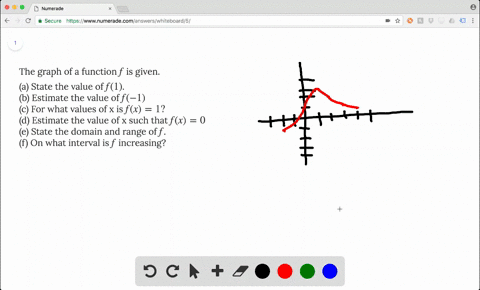Enroll in one of our FREE online STEM summer camps. Space is limited so join now!View Summer Courses### The graph of a function $f$ is given. (a) S…

03:25Oregon State University

Need more help? Fill out this quick form to get professional live tutoring.

Get live tutoring
Problem 2

If $f(x) = \frac{x^2-x}{x-1}$ and $g(x) = x$ is it true that $f = g$?

$f \neq g$ because their domain is not equal.

## Discussion

You must be signed in to discuss.
Asif K.
September 29, 2019
Why o divided by zero is undefined??

## Video Transcript

here we have f of X, and we have G of X, and we want to know if they're equal. So chances are what you're going to do is look at f of X and realize that you can factor the numerator. You can factor in X out of both terms and you have X Times X minus one over X minus one and then you're likely to think, Well, why don't I just cancel the X minus ones? And then I'll have left his ex and it's just f of X equals X? Well, that's only partially true. Go back to the original function and think about the domain. Let's think about the domain of F of X. You can't have zero for your denominator, so that means that X cannot be won. Let's think about the domain of G of X G of X is just a polynomial function, and all real numbers can be and put it into their, and an output of real number will result. So the domain of G is all real numbers. So from that piece of information alone, we know that they're not the same function. And if you want to consider the graph, consider the graph of G. It's just going to be the line with a slope of one and a Y intercept of zero. So then how would the graph of F B any different? Well, since you can't have X equals one, you can't have the 10.11 that would be on G. And so you would have a whole at 11 And other than that, you would have something that looks like G. So these are not the same.